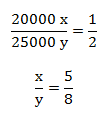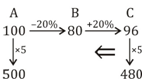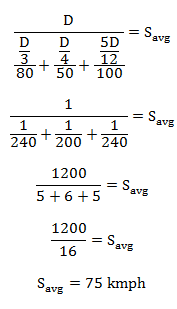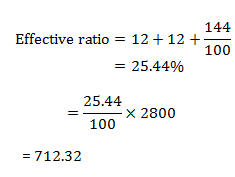# SSC Quantitative Aptitude Questions (Day-70)

Dear Aspirants, you can find the Quantitative Aptitude questions with detailed explanations for the SSC exams. Nowadays the competitive level of the exam has been increasing consistently. Due to the great demand for the government job, the level of the toughness reached greater. Candidates have to enhance the preparation process in order to drive in the right path. It doesn’t need to clear the prescribed cutoff. You must have to score good marks more than the cut off marks to get into the final provisional list. Here we have updating the Quantitative Aptitude questions with detailed explanations on a daily basis. You can practice with us and measure your level of preparation. According to that you can sculpt yourself in a proper way. SSC aspirants kindly make use of it and grab your success in your career.

Start Quiz

1) A started a business with Rs 20000 and B joined after some time with Rs 25000. If A and B share the profit in the ratio of 1 : 2 respectively, then whatis the ratio of the time period of investment for A and B respectively?

(a) 1 : 2

(b) 5 : 6

(c) 5 : 8

(d) 5 : 7

2) The average of 6 consecutive even numbers is 25. If the next even number is also considered, then what is the new average?

(a) 27

(b) 25

(c) 26

(d) 28

3) There is group of 8 teachers. One teacher leaves the group and a new teacher joins the group. Due to this, the average age of teachers becomes sameas the average 2 years ago. If the member who left was aged 42. Then what is the age (in years) of new teacher?

(a) 22

(b) 28

(c) 24

(d) 26

4) A purchased an article and sold it to B at a loss of 20% and B sold it to C at a gain of 20%. C purchases the article for Rs 480. For what price (in Rs) Ahas purchased the article?

(a) 500

(b) 450

(c) 420

(d) 480

5) 20% of cost price is equal to 30% of the selling price. What is the loss percentage?

(a) 33.33

(b) 50

(c) 20

(d) 25

6) Salary of A is 20% more than B and salary of B is 18% more than C. The salary of C is (approximately) how much percent less than A?

(a) 29.3%

(b) 32.5%

(c) 26.7%

(d) 28.1%

7)  Price of sugar increases by 30%. If expenditure increases by only 10%, then by how much percent consumption should decrease?

(a) 14.29

(b) 15.38

(c) 13.68

(d) 16.54

8) Amit goes to his office by car at the speed of 80 km/hr and reaches 15 minutes earlier. If he goes at the speed 60 km/hr, he reaches 15 minutes late.What will be the speed (in km/hr) of the car to reach on time?

(a) 26 2/7

(b) 67 4/7

(c) 68 4/7

(d) 69 4/7

9) One third of a certain journey is covered at the speed of 80 km/hr, one fourth of the journey at the speed of 50 km/hr and the rest at the speed of 100km/hr. what will be the average speed(in km/hr) for the whole journey?

(a) 75

(b) 67

(c) 66.66

(d) 76.66

10) What is the compound interest (in Rs) on a principle sum of Rs 2800 for 2 years at the rate of 12% per annum?

(a) 687.18

(b) 634.46

(c) 712.32

(d) 568.68

A : 20000 x

B : 25000 y

Ratio of investment = Ratio of Profit sharedAverage of 6 consecutive no.= 25 = Average of 3rd& 4th No.

If nos. are odd and they are in A.P.

Then middle term is the Average/mean ,which is 4th term.

3rd term = 25 – 1 = 24

4th term = 25 + 1 = 26

Average becomes equal to the Avg. 2 years before means it is reduced by 2 years. Hence there will be a decrease of 2 years from each person , 28 = 16

Age of the new teacher

= 42 – 16

= 26 yrs.20 C.P. = 30 S.P.

SP/CP = 2/3

Hence loss % = 33.33%

C        B          A

100 → 118 → 141.6

C is % less than A = (1–100/141.6) × 100% = 29.3%

Let the price of sugar is x

New price of sugar is 1.3x

Quantity of sugar purchased ‘y’

New quantity (y – k)

(1.1) xy = (1.3x) (y – k)

(1.1) xy = x1.3 x (y –k)

(y – k)/y = 0.8461 × 100%

= 84.61%

Hence (100 – 84.61) = 15.38%

Speed becoming → 3/4 s

Hence time taken to travel will become 4/3 t

Time increase 1/3 t = 30

t = 90 min

Dist. = 1.5 × 80 = 120 km

New time to reach is 105 min 1¾ hr.

= (120 × 4)/7

= 480/7 = 684/7

OR

80 (t – 1/4 ) = 60 (t +1/4 )

By solving t = 7/4

Distance= 60 × 2 = 120

Speed = 120 × 4/7 = 480/7 = 68 4/7